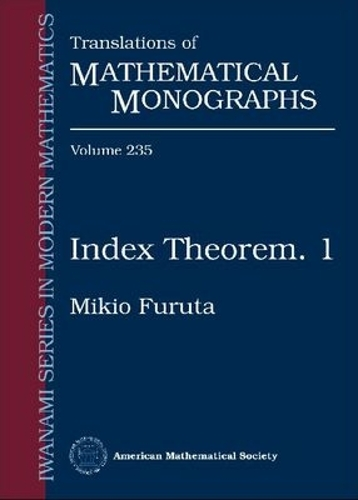•Index Theorem 1 - Translations of Mathematical Monographs (Paperback)

£45.95
Paperback Published: 30/11/2007
• We can order this

Usually dispatched within 3 weeks

The Atiyah-Singer index theorem is a remarkable result that allows one to compute the space of solutions of a linear elliptic partial differential operator on a manifold in terms of purely topological data related to the manifold and the symbol of the operator. First proved by Atiyah and Singer in 1963, it marked the beginning of a completely new direction of research in mathematics with relations to differential geometry, partial differential equations, differential topology, K-theory, physics, and other areas.

Publisher: American Mathematical Society
ISBN: 9780821820971
Weight: 267 g